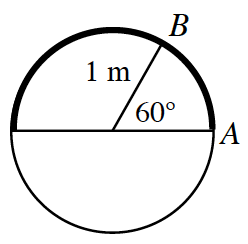### Home > PC3 > Chapter 1 > Lesson 1.2.3 > Problem1-102

1-102.The circle at right has a radius of one meter.

1. How many degrees are in a half circle?

It is half of $360°$.

2. What is the length of the arc that covers half of the circle?

The circumference of the circle is $2\pi(1\text{m})$. What is half of the circumference?

3. Using exact values, what is the length of  $\overparen {\textit{AB}}$? You will have $\pi$ as part of your answer.

What part of the circumference will $60°$ have?

What is $\frac{1}{6}$ of the circumference?